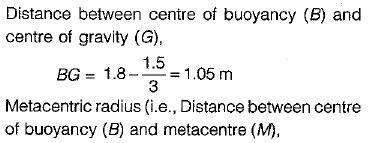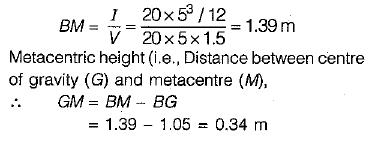Courses

# Test: Buoyancy And Floatation

## 10 Questions MCQ Test Topicwise Question Bank for GATE Civil Engineering | Test: Buoyancy And Floatation

Description
This mock test of Test: Buoyancy And Floatation for Civil Engineering (CE) helps you for every Civil Engineering (CE) entrance exam. This contains 10 Multiple Choice Questions for Civil Engineering (CE) Test: Buoyancy And Floatation (mcq) to study with solutions a complete question bank. The solved questions answers in this Test: Buoyancy And Floatation quiz give you a good mix of easy questions and tough questions. Civil Engineering (CE) students definitely take this Test: Buoyancy And Floatation exercise for a better result in the exam. You can find other Test: Buoyancy And Floatation extra questions, long questions & short questions for Civil Engineering (CE) on EduRev as well by searching above.
QUESTION: 1

### A floating body is in stable equilibrium when

Solution:

Floating body will be under stable equilibrium when metacentre is positive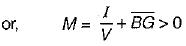QUESTION: 2

Solution:
QUESTION: 3

### A small plastic boat loaded with nuts and bolts is floating in a bath tub. if the cargo is dumped into the water, allowing the boat to float empty, then the water level in the tub will

Solution:
QUESTION: 4

The line of action of the buoyant force acts through the

Solution:
QUESTION: 5

A body is floating as shown in the given figure. The centre of buoyancy, centre of gravity and metacentre are labelled respectively as B, G and M. The body is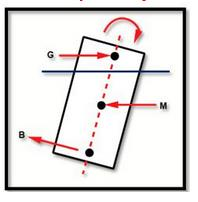Solution:

Since metacentre is below centre of gravity the body is in unstable equilibrium in rotation.

QUESTION: 6

A metal cube of size 15 cm x 15 cm x 15 cm and specific gravity 8.6 is submerged in a twolayered liquid, the bottom layer being mercury and the top layer being water. The percentage of the volume of the cube remaining above the interface will be, approximately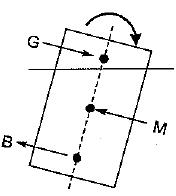Solution: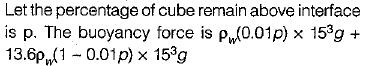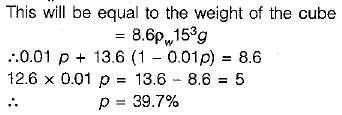QUESTION: 7

The centre of gravity of the volume of liquid displaced is called

Solution:
QUESTION: 8

When a block of ice floating on,water in a container melts, the level of water in the container

Solution:
QUESTION: 9

A metal block is thrown into a deep lake. As it sinks deeper in water, the buoyant force acting on it​

Solution:
QUESTION: 10

A rectangular floating body is 20 m long and 5 m wide. The water line is 1.5 m above the bottom. If the centre of gravity is 1.8 m from the bottom, then its metacentric height will be approximately

Solution: